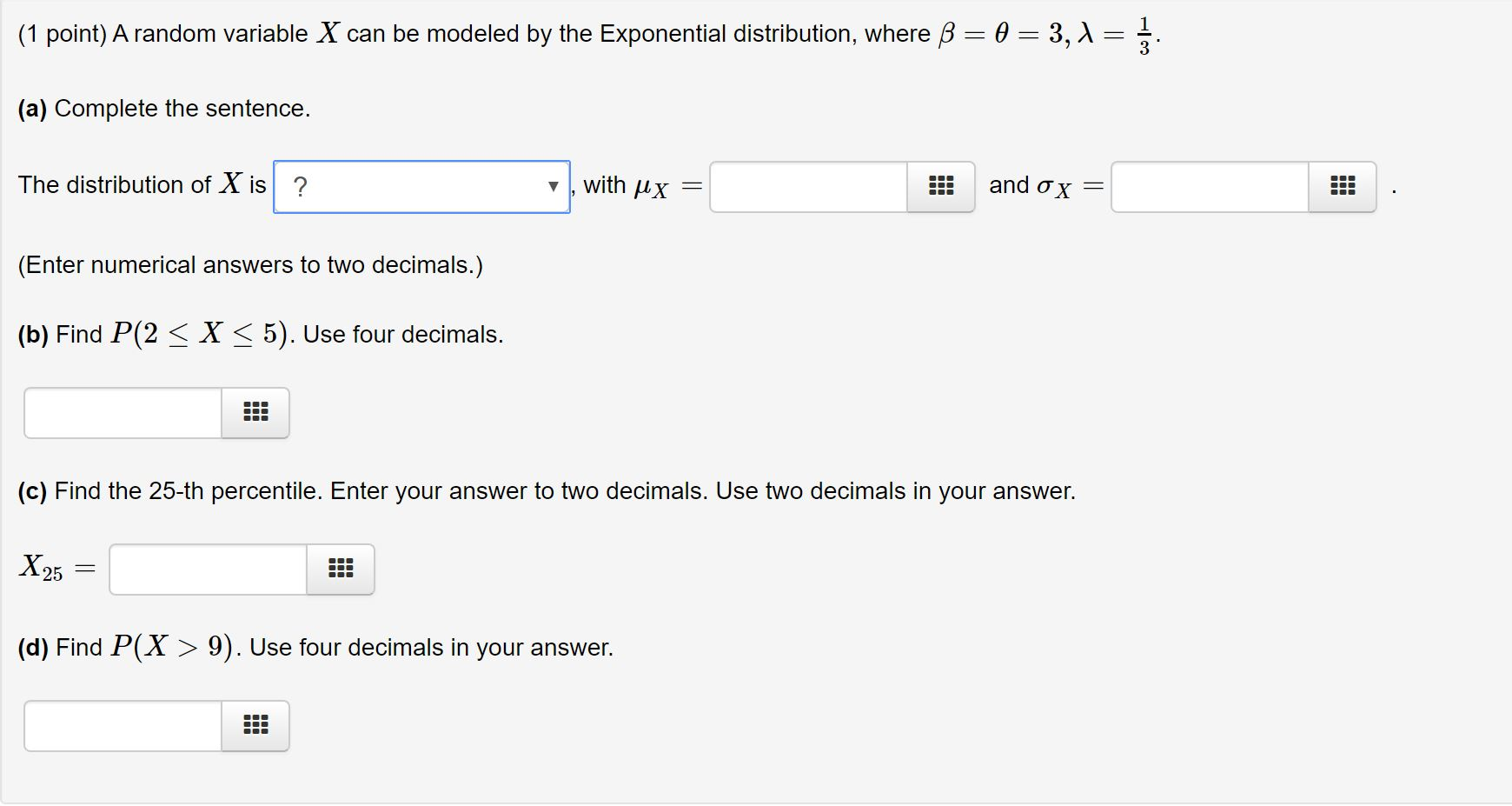ZWU3SU The Asker · Probability and StatisticsTranscribed Image Text: (1 point) A random variable X can be modeled by the Exponential distribution, where ß= 0 = 3,1=1 (a) Complete the sentence. The distribution of X is with px = !!! and ox = (Enter numerical answers to two decimals.) (b) Find P(2 < X <5). Use four decimals. (c) Find the 25-th percentile. Enter your answer to two decimals. Use two decimals in your answer. X 25 = (d) Find P(X > 9). Use four decimals in your answer.
More
Transcribed Image Text: (1 point) A random variable X can be modeled by the Exponential distribution, where ß= 0 = 3,1=1 (a) Complete the sentence. The distribution of X is with px = !!! and ox = (Enter numerical answers to two decimals.) (b) Find P(2 < X <5). Use four decimals. (c) Find the 25-th percentile. Enter your answer to two decimals. Use two decimals in your answer. X 25 = (d) Find P(X > 9). Use four decimals in your answer.